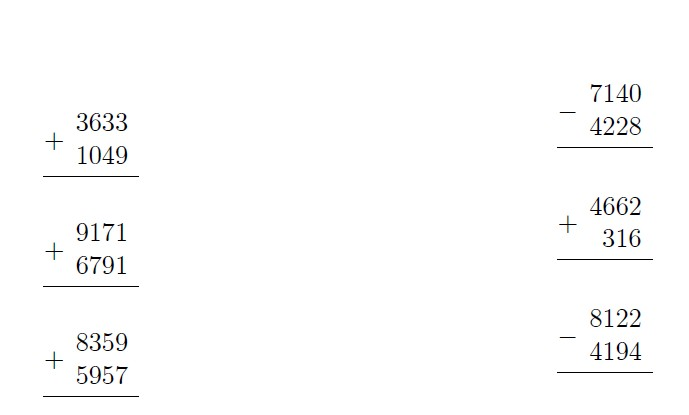# LaTex: Exercise

In order to give the oldest child examples of addition and subtraction in a column, I wrote a Groovy script that generates LaTeX with examples.
The goal was to practice grooves, well, tired of writing examples by hand.

Previously, he did not write anything at the lathe, but he knew what it was and why it was needed from Univer.
About two hours fucked with how to make the desired type of column on LaTeX.
To work with LaTeX, I installed the appropriate packages from CygWin.

Here is the script, I hope someone comes in handy:

//////////////////////////////////////////////////// int n = 30 def f1 = new File("d:\\temp\\ex.tex") def f2 = new File("d:\\temp\\sol.tex") ///////////////////////////////////////////////////// def r = new Random(System.currentTimeMillis()) f1.delete(); f2.delete(); def header = """ \\documentclass[a4paper,12pt,twocolumn]{article} \\begin{document} \n""" f1<< header f2<< header n.times { int a = r.nextInt(10000) int b = r.nextInt(10000) boolean plus = r.nextBoolean() if (a int c = plus?a+b:a-b def sign = plus?"+":"-" f1<< """\$\$ \\frac{$sign \\begin{array}{rcc}$a \\\\ $b \\end{array}}{} \$\$\n""" f2<< """\$\$\\frac{$sign \\begin{array}{rcc} $a \\\\$b \\end{array}}{$c} \$\\$\n""" } def footer = """ \\end{document} \n""" f1<
 f2<

Here's what it looks like:PS The most suitable blog, it seems, is development. Or transfer to some other?